# Quick Hit: Above the Fold; Hard wrapping text at ‘n’ characters

Despite being on holiday I’m getting in a bit of non-work R coding since the fam has a greater ability to sleep late than I do. Apart from other things I’ve been working on a PR into {lutz}, a package by @andyteucher that turns lat/lng pairs into timezone strings.

The package is super neat and has two modes: “fast” (originally based on a {V8}-backed version of @darkskyapp’s tzlookup javascript module) and “accurate” using R’s amazing spatial ops.

I ported the javascript algorithm to C++/Rcpp and have been tweaking the bit of package helper code that fetches this: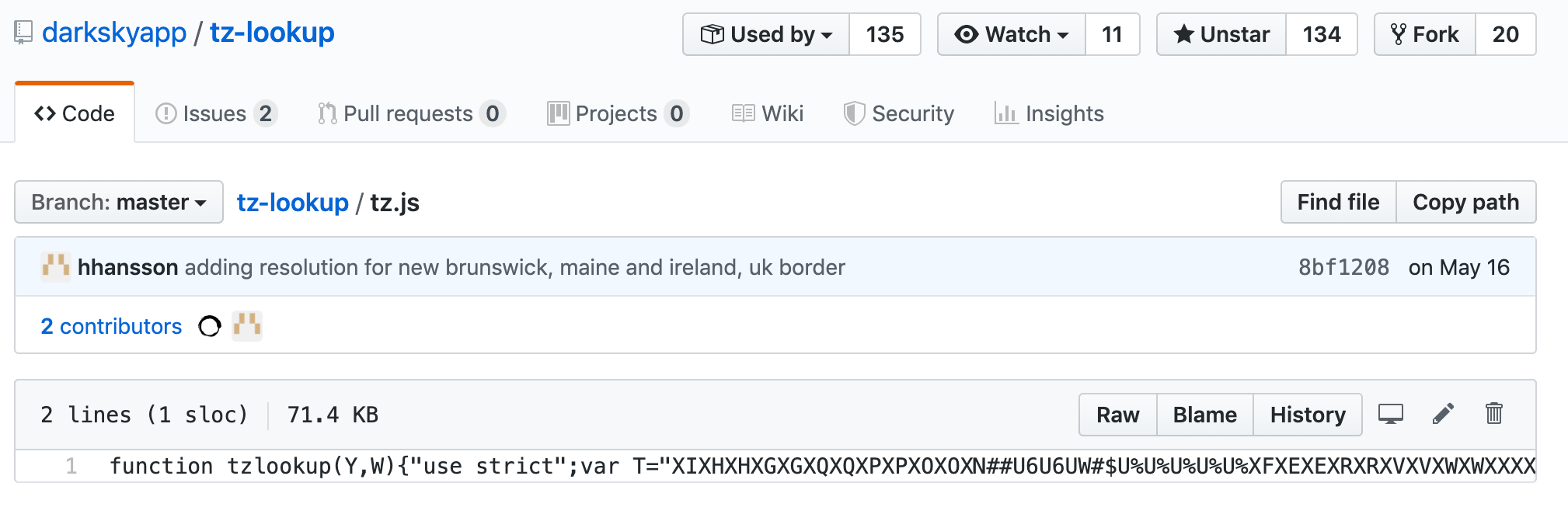and extracts the embedded string tree and corresponding timezones array and turns both into something C++ can use.

Originally I just made a header file with the same long lines: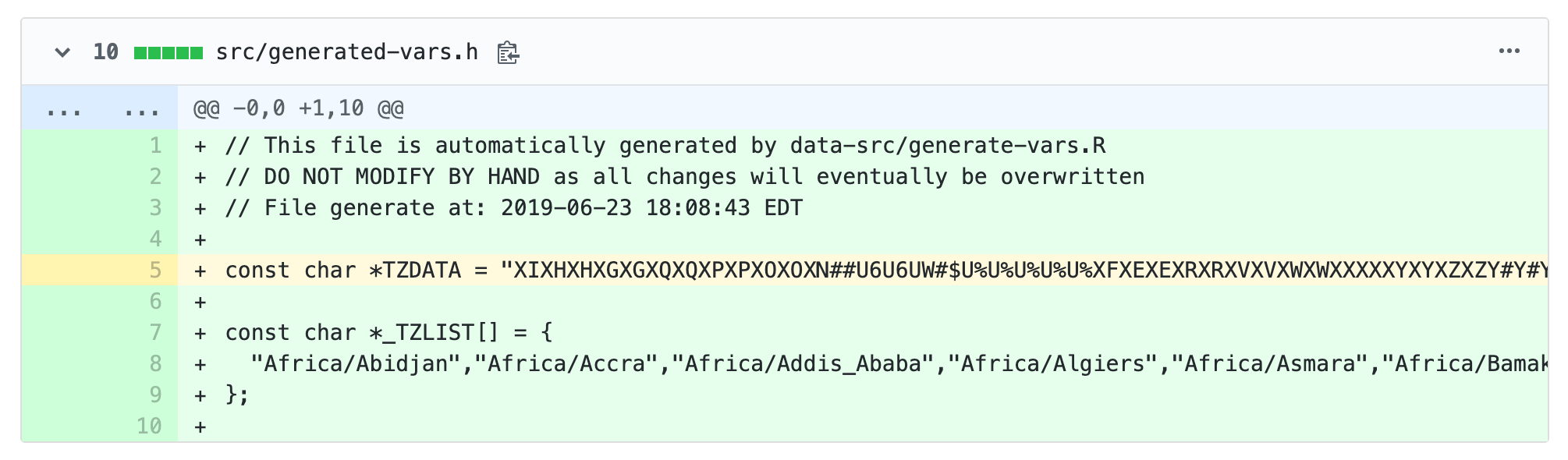but that’s icky and fairly bad form, especially given that C++ will combine adjacent string literals for you.

The `stringi::stri_wrap()` function can easily take care of wrapping the time zone array elements for us: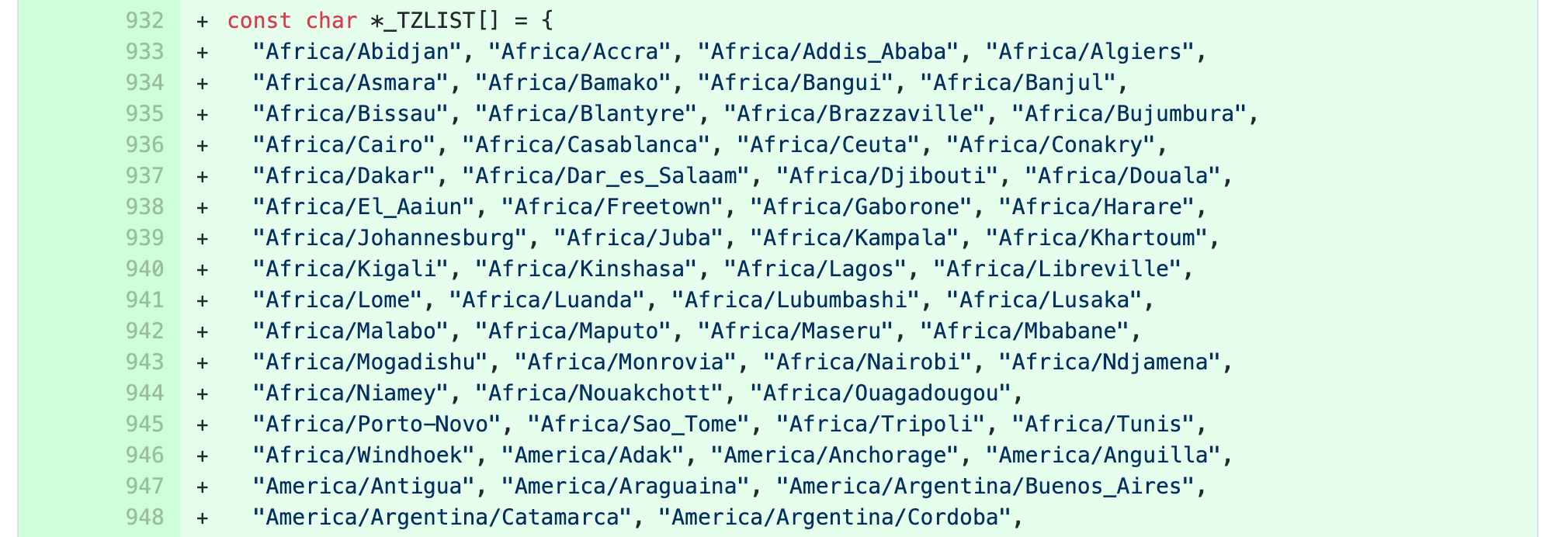but, I also needed the ability to hard-wrap the encoded string tree at a fixed width. There are lots of ways to do that, here are three of them:

``````library(Rcpp)
library(stringi)
library(tidyverse)
library(hrbrthemes)
library(microbenchmark)

sourceCpp(code = "
#include <Rcpp.h>

// [[Rcpp::export]]
std::vector< std::string > fold_cpp(const std::string& input, int width) {

int sz = input.length() / width;

std::vector< std::string > out;
out.reserve(sz); // shld make this more efficient

for (unsigned long idx=0; idx<sz; idx++) {
out.push_back(
input.substr(idx*width, width)
);
}

if (input.length() % width != 0) out.push_back(input.substr(width*sz));

return(out);
}
")

fold_base <- function(input, width) {

vapply(
seq(1, nchar(input), width),
function(idx) substr(input, idx, idx + width - 1),
FUN.VALUE = character(1)
)

}

fold_tidy <- function(input, width) {

map_chr(
seq(1, nchar(input), width),
~stri_sub(input, .x, length = width)
)

}
``````

(If you know of a package that has this type of function def leave a note in the comments).

Each one does the same thing: move `n` sequences of `width` characters into a new slot in a character vector. Let’s see what they do with this toy long string example:

``````(src <- paste0(c(rep("a", 30), rep("b", 30), rep("c", 4)), collapse = ""))
##  "aaaaaaaaaaaaaaaaaaaaaaaaaaaaaabbbbbbbbbbbbbbbbbbbbbbbbbbbbbbcccc"

for (n in c(1, 7, 30, 40)) {

print(fold_base(src, n))
print(fold_tidy(src, n))
print(fold_cpp(src, n))
cat("\n")

}
##   "a" "a" "a" "a" "a" "a" "a" "a" "a" "a" "a" "a" "a" "a" "a" "a" "a"
##  "a" "a" "a" "a" "a" "a" "a" "a" "a" "a" "a" "a" "a" "b" "b" "b" "b"
##  "b" "b" "b" "b" "b" "b" "b" "b" "b" "b" "b" "b" "b" "b" "b" "b" "b"
##  "b" "b" "b" "b" "b" "b" "b" "b" "b" "c" "c" "c" "c"
##   "a" "a" "a" "a" "a" "a" "a" "a" "a" "a" "a" "a" "a" "a" "a" "a" "a"
##  "a" "a" "a" "a" "a" "a" "a" "a" "a" "a" "a" "a" "a" "b" "b" "b" "b"
##  "b" "b" "b" "b" "b" "b" "b" "b" "b" "b" "b" "b" "b" "b" "b" "b" "b"
##  "b" "b" "b" "b" "b" "b" "b" "b" "b" "c" "c" "c" "c"
##   "a" "a" "a" "a" "a" "a" "a" "a" "a" "a" "a" "a" "a" "a" "a" "a" "a"
##  "a" "a" "a" "a" "a" "a" "a" "a" "a" "a" "a" "a" "a" "b" "b" "b" "b"
##  "b" "b" "b" "b" "b" "b" "b" "b" "b" "b" "b" "b" "b" "b" "b" "b" "b"
##  "b" "b" "b" "b" "b" "b" "b" "b" "b" "c" "c" "c" "c"
##
##   "aaaaaaa" "aaaaaaa" "aaaaaaa" "aaaaaaa" "aabbbbb" "bbbbbbb"
##   "bbbbbbb" "bbbbbbb" "bbbbccc" "c"
##   "aaaaaaa" "aaaaaaa" "aaaaaaa" "aaaaaaa" "aabbbbb" "bbbbbbb"
##   "bbbbbbb" "bbbbbbb" "bbbbccc" "c"
##   "aaaaaaa" "aaaaaaa" "aaaaaaa" "aaaaaaa" "aabbbbb" "bbbbbbb"
##   "bbbbbbb" "bbbbbbb" "bbbbccc" "c"
##
##  "aaaaaaaaaaaaaaaaaaaaaaaaaaaaaa" "bbbbbbbbbbbbbbbbbbbbbbbbbbbbbb"
##  "cccc"
##  "aaaaaaaaaaaaaaaaaaaaaaaaaaaaaa" "bbbbbbbbbbbbbbbbbbbbbbbbbbbbbb"
##  "cccc"
##  "aaaaaaaaaaaaaaaaaaaaaaaaaaaaaa" "bbbbbbbbbbbbbbbbbbbbbbbbbbbbbb"
##  "cccc"
##
##  "aaaaaaaaaaaaaaaaaaaaaaaaaaaaaabbbbbbbbbb"
##  "bbbbbbbbbbbbbbbbbbbbcccc"
##  "aaaaaaaaaaaaaaaaaaaaaaaaaaaaaabbbbbbbbbb"
##  "bbbbbbbbbbbbbbbbbbbbcccc"
##  "aaaaaaaaaaaaaaaaaaaaaaaaaaaaaabbbbbbbbbb"
##  "bbbbbbbbbbbbbbbbbbbbcccc"
``````

So, we know they all work, which means we can take a look at which one is faster. Let’s compare folding at various widths:

``````map_df(c(1, 3, 5, 7, 10, 20, 30, 40, 70), ~{
microbenchmark(
base = fold_base(src, .x),
tidy = fold_tidy(src, .x),
cpp = fold_cpp(src, .x)
) %>%
mutate(width = .x) %>%
as_tibble()
}) %>%
mutate(
width = factor(width,
levels = sort(unique(width)),
ordered = TRUE)
) -> bench_df

ggplot(bench_df, aes(expr, time)) +
ggbeeswarm::geom_quasirandom(
aes(group = width, fill = width),
groupOnX = TRUE, shape = 21, color = "white", size = 3, stroke = 0.125, alpha = 1/4
) +
scale_y_comma(trans = "log10", position = "right") +
coord_flip() +
guides(
fill = guide_legend(override.aes = list(alpha = 1))
) +
labs(
x = NULL, y = "Time (nanoseconds)",
fill = "Split width:",
title = "Performance comparison between 'fold' implementations"
) +
theme_ft_rc(grid="X") +
theme(legend.position = "top")
````````````ggplot(bench_df, aes(width, time)) +
ggbeeswarm::geom_quasirandom(
aes(group = expr, fill = expr),
groupOnX = TRUE, shape = 21, color = "white", size = 3, stroke = 0.125, alpha = 1/4
) +
scale_x_discrete(
labels = c(1, 3, 5, 7, 10, 20, 30, 40, "Split/fold width: 70")
) +
scale_y_comma(trans = "log10", position = "right") +
scale_fill_ft() +
coord_flip() +
guides(
fill = guide_legend(override.aes = list(alpha = 1))
) +
labs(
x = NULL, y = "Time (nanoseconds)",
fill = NULL,
title = "Performance comparison between 'fold' implementations"
) +
theme_ft_rc(grid="X") +
theme(legend.position = "top")
``````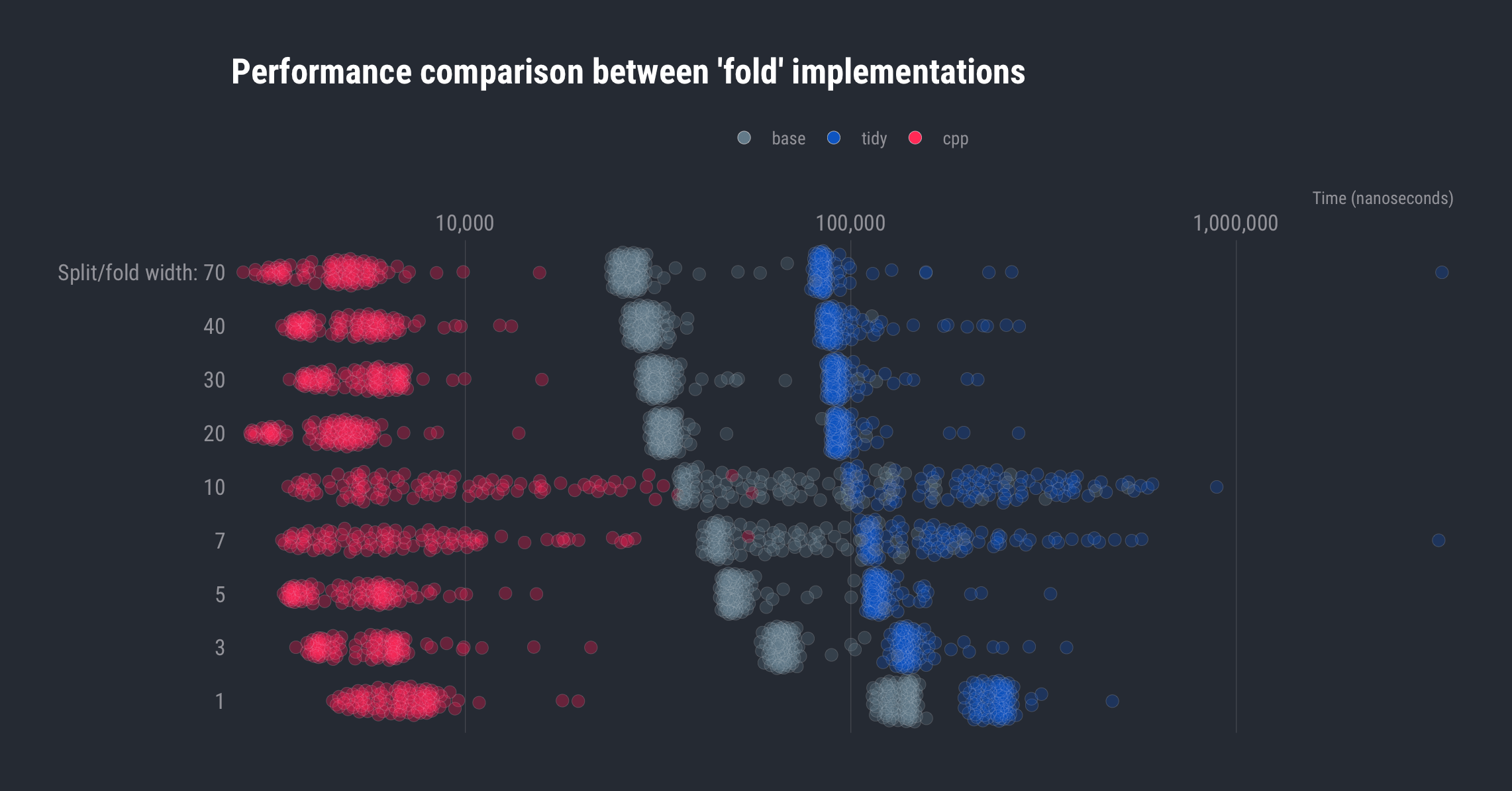The Rcpp version is both faster and more consistent than the other two implementations (though they get faster as the number of string subsetting operations decrease); but, they’re all pretty fast. For an infrequently run process, it might be better to use the base R version purely for simplicity. Despite that fact, I used the Rcpp version to turn the string tree long line into: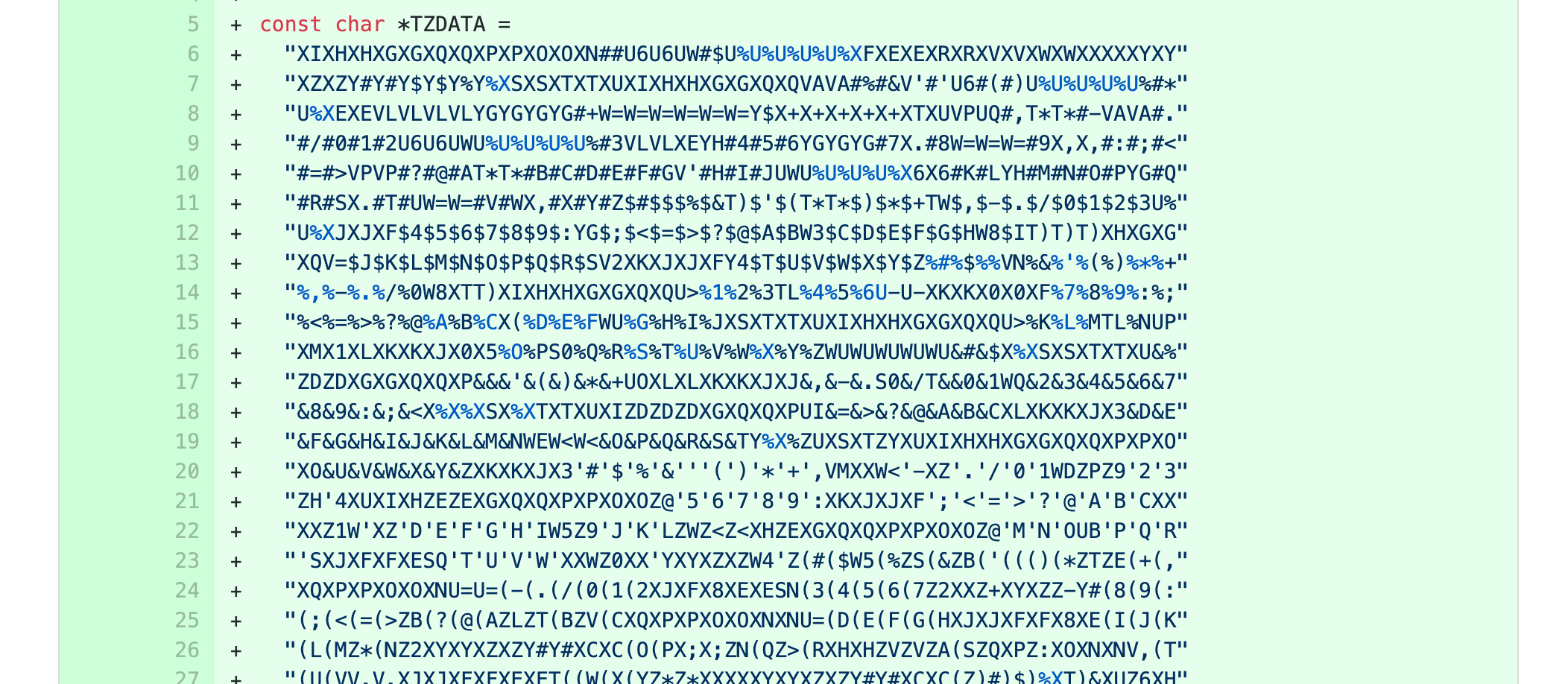### FIN

If you have need to “fold” like this how do you currently implement your solution? Found a bug or better way after looking at the code? Drop a note in the comments so you can help others find an optimal solution to their own ‘fold’ing problems.Amazon Author Page

## 3 Comments →Quick Hit: Above the Fold; Hard wrapping text at ‘n’ characters

1.Richard An

Hello,

I got an error message as bellow:

Error in scale_y_comma(trans = “log10”, position = “right”) :
could not find function “scale_y_comma”

1.hrbrmstr

Thx for identifying that. I just added the missing `library(hrbrthemes)` to the post.

2.Marek Szatkowski

1st: `stringi` functions are vectorized so no need for map_chr:

``` fold_tidy <- function(input, width) stri_sub(input, seq(1, nchar(input), width), length = width) ```

which makes it as efficient as base sollution.

``` fold_tidy <- function(input, width) stri_sub(input, seq.int(1L, nchar(input), width), length = width) ```